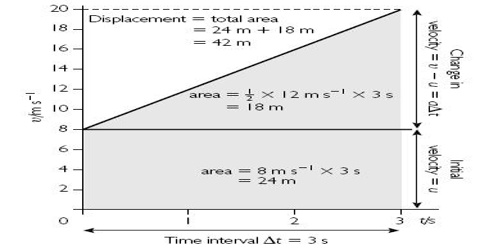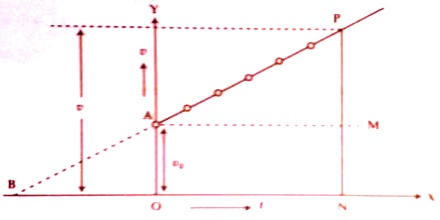# Derivation of Equations of Motion with the help of Graphs

Derivation of Equations of motion with the help of graphs

(a) Graph in case of the equation, v = v0 + at

In this equation, there are two variables— one is t and the other one is v. A graph is drawn taking t on the X-axis and v on the Y-axis [Fig]. In the figure, normal PN is drawn from point P to Y-axis. Let consider that the final velocity at time t = v = OY. Now OY = OA + AY i.e., v = v0 + at.Here, slope, a = PM/AM = AY/AM = AY/t

The equation v = v0 + at is obtained from the graph.

In case of motion of a body from rest, v0 = 0, a = constant.

So, v = 0 + constant x t

so, v ∞ t

That means, velocity is proportional to time.

(b) Graph for the equation, s = v0t + ½ at2

In figure, graph of ‘v’ versus ‘t’ is a straight line.

In the figure PN ┴ OX; AM ┴ PN.

Let initial velocity = v0, uniform acceleration = a, ON = t and distance travelled in time t = s.

Now, s = Area of OAPN

= area of the rectangle OAMN + area of the triangle AMP

= OA x ON + ½ AM PM

= v0t + ½ AM PM

Again, slope ‘a’ PM/AM = PM/t

so, PM = at

and, s = v0t + ½ t.at = v0t + ½ at2

So, the equation can be presented in graph.

In case of a body moving with uniform acceleration from rest, then,

s = 0 x t + ½ constant x t2

or, s = constant x t2

or, s ∞ t2

That means, displacement is proportional to time.